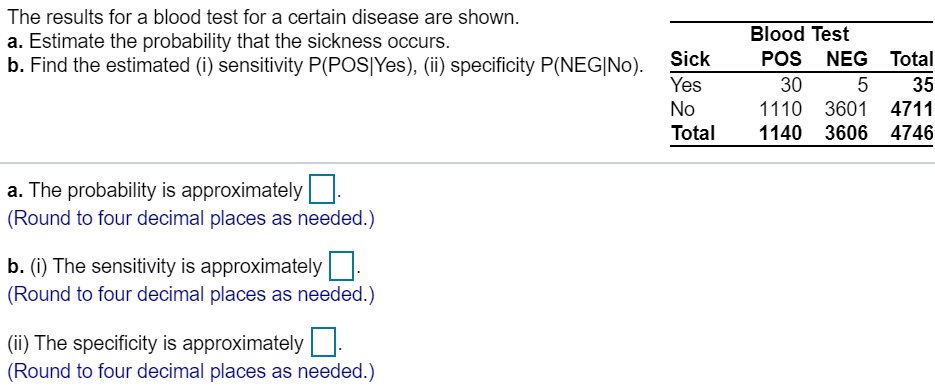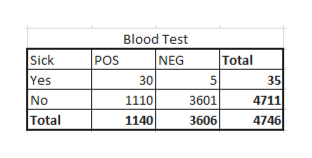# The results for a blood test for a certain disease are shown.Blood Testa. Estimate the probability that the sickness occurs.b. Find the estimated (i) sensitivity P(POSIYes), (ii) specificity P(NEG|No).POSNEGTotalSickYes30535No1110 360147111140 3606Total4746a. The probability is approximately(Round to four decimal places as needed.)b. (i) The sensitivity is approximately(Round to four decimal places as needed.)(i) The specificity is approximately(Round to four decimal places as needed.)

Questionhelp_outlineImage TranscriptioncloseThe results for a blood test for a certain disease are shown. Blood Test a. Estimate the probability that the sickness occurs. b. Find the estimated (i) sensitivity P(POSIYes), (ii) specificity P(NEG|No). POS NEG Total Sick Yes 30 5 35 No 1110 3601 4711 1140 3606 Total 4746 a. The probability is approximately (Round to four decimal places as needed.) b. (i) The sensitivity is approximately (Round to four decimal places as needed.) (i) The specificity is approximately (Round to four decimal places as needed.) fullscreen
check_circleExpert Solution
Step 1

We are given,

The results of blood test for a certain disease:help_outlineImage TranscriptioncloseBlood Test NEG Sick Yes POS Total 30 1110 35 5 No Total 3601 4711 1140 4746 3606 fullscreen
Step 2

a)We have to find the probability that the sickness occurs.

That is to find P(Sick) = ….?

Step 3

b)

i)We have to find Sensitivity. That is, P(POS|Yes) =…...

### Want to see the full answer?

See Solution

#### Want to see this answer and more?

Solutions are written by subject experts who are available 24/7. Questions are typically answered within 1 hour*

See Solution
*Response times may vary by subject and question
Tagged in

### Statistics